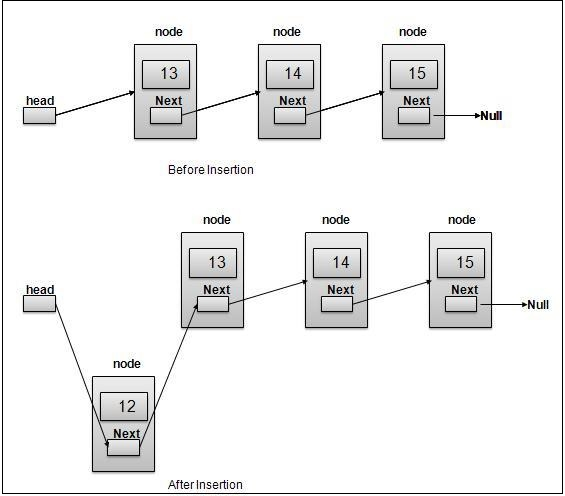We need to create a function insert(data, position) that inserts data at given position in the linked list. We'll perform the following steps −

• Create a new Node
• Check if the list is empty. If it then adds the node to head and return.
• If not, then we'll iterate to the position we want to insert it to using currElem. We iterate a linked list by making currElem equal to currElem.next.
• Then we'll make node point to the next node in the list. This is to keep track of the rest of the list.
• Finally, we break the link from currElem to rest of the list and make it point to our created node. Now the node is in the list at the given position.

Here is an illustration of the same −Now let's have a look at how we'll implement this −

## Example

insert(data, position = this.length) {
let node = new this.Node(data);
this.length++;
}
let iter = 1;
while (currNode.next != null && iter < position) {
currNode = currNode.next; iter++;
}
node.next = currNode.next;
currNode.next = node;
this.length++;
return node;
}

Note that we've given position as the last element. This is because if you don’t provide a position, it'll be inserted at the end by default.

You can test this using −

## Example

let list = new LinkedList();
list.insert(10);
list.insert(20);
list.insert(30);
list.insert(15, 2);
list.display();

## Output

This will give the output −

10 ->
30 ->
15 ->
20 ->

As we can see all the elements are in the order we intended. We tried inserting 15 at the position after 2.